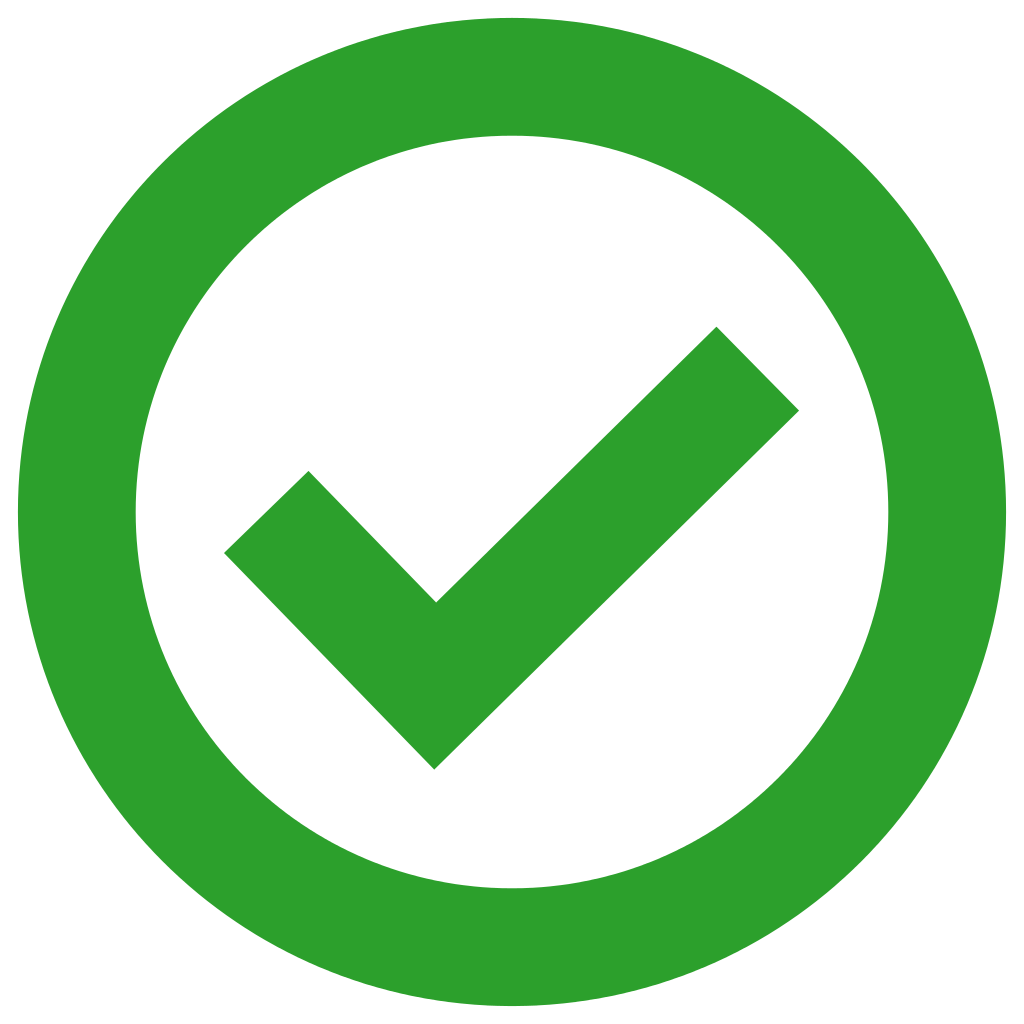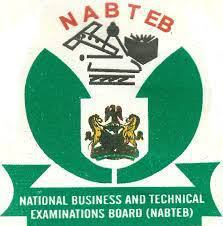## NABTEB 2019 CHEMISTRY PRACTICAL ANSWER(1a)
A = 20.0gdm^-3 NaHCO4
B = 5.60gdm^-3 KOH

Equation of reaction: 2NaHSO4(aq) + KOH(aq) = NaSO4(aq) + K2SO4(aq) + 2H2O(l)

Final burette reading (cm^3): 20.45, 18.93, 20.02

Initial burette reading (cm^3): 0.00, 0.00, 1.00

Volume of A used (cm^3) : 20.45, 18.93, 19.02

Average volume of Acid used =18.93+19.02/2
=37.95/2
Average volume of Acid used =18.98cm^3

(1bi)
Concentration of moldm^-3
Molarity = Mass concentration /molar mass
Molarity=5.60/56
Molarity= 0.10moldm^-3

(1bii)
Concentration in A moldm^-3
Cava/CbVb =na/nb
Ca=CbVbna / Vanb

=0.100*200*2/18.98*1
Ca = 5/18.98
Ca = 0.263moldm^-3
Concentration of A moldm^-3 is 0.263moldm^-3

(1biii)
Percentage of NaHSO4 in NaHSO4
NaOH(aq) + H2SO4 (aq) —> NaHSO4(aq) + H2O(l)

Molar mass of NaHSO4 = 104gmol^-1
% of sodium tetraoxosulphate(vi) =103/104*100/1
= 99.038
Percentage of sodium tetraoxosulphate(vi)= 99.04%
=============================

NOTE: DRAW TABLE HERE, TEST comes first, OBSERVATION follows, then INFERENCE

(2a)
Test: Sample C + 10cm of distilled water

Observation: Dissolves to form a colourless solution

Inference: C is a soluble salt of Na^+

(2aii)
Test: Sample C solution + litmus paper

Observation: It turns most blue litmus paper red

Inference: Acid gas present in salt sample C

(2aiii)
Test: 2cm^3 portion of sample + Bacl(aq) + Excess HCL(aq) + Warm

Observation: White precipitate formed which is soluble in excess dilute HCL(aq). A colourless gas with an irritating odour evolved which turns acidified K2Cr2O7 solution from orange to green

Inference; SO4^2- and SO3^2- is present while SO3^2- is confirmed. Also SO2 gas confirmed from SO3^2-

(2b)
Test: 2cm^3 portion of sample D + HCl(aq)

Observation; A reddish-brown precipitate is formed

Inference; Fe^3+ present

(2bi)
Test: 2cm^3 portion of sample D + HCL(aq) + 5cm^3 of solution C + Heat gently to boil then allowed to cool under water

Observation; White precipitate is formed which is insoluble in excess HCL. The precipitate turn brown at the upper part of the tube

CHECK OUT:  LIST OF OUR EXAM RUNS PACKAGES AND THEIR EXPLANATIONS

Inference; Fe^2+ present, SO3^2- hence Fe^2+ oxidized to Fe^3+

(2bii)
Test; 2cm^3 portion of the coded solution in (2bi) + NH3(aq) + drops + excess

Observation: White precipitate formed, insoluble in excess NH3 solution

Inference; Fe^3+ present

(2biii)
Test; Sample in 2bi in test tube for 5minutes

Observation: Precipitate turned brown

Inference; Fe^3+ Confirmed
=============================

(3ai)
Substance of E + iodine solution gives a blue black colouration , hence E is a starch solution. E is boiled with HCL(aq) hence starch is hydrolysed, so visible reaction sample is Glucose or sucrose ie F=Glucose , G is zymase which convert Glucose to ethanol and CO2, hence H is carbon dioxide gas (CO2). Ethanol treated with propanoic acid ( C3H7COOH ) to obtain C3H7COOC2H5 (propylpropanoate) and water.
(3aii)
C3H7COOH(aq) + C2H5OH(aq) —-> C3H7COOC2H5(aq) (propylpropanoate) + H2O(l) (water)
(a) The concentration of propanoic acid used
(b) The functional group of the reactant as -COOH and -OH
(3aiii)
C3H7COOH(aq) + C2H5OH(aq) —-> C3H7COOC2H5(aq) + H2O(l)
(3b)
A gas jar is prepared containing water for upward collection of gas or downward collection
============================
COMPLETED
============================Join Our Telegram Channel |Whatsapp Our Admins: Click Any Name Mr.Paul or Mr.Henry

### Comment

Posted by on 14th May 2019.

Tags:

Categories: NABTEB

## 2 Responses

1. I want you Guys to be dropping the Answer together with the Screenshot of the Exam Questions. I think it’ll be so Nice, thanks

by Abdulrahman Haladu on May 14, 2019

2. And please you Guys you’re helping us, we need you help again to be posting us the Expo even before 2 hours to Exams.

by Abdulrahman Haladu on May 14, 2019